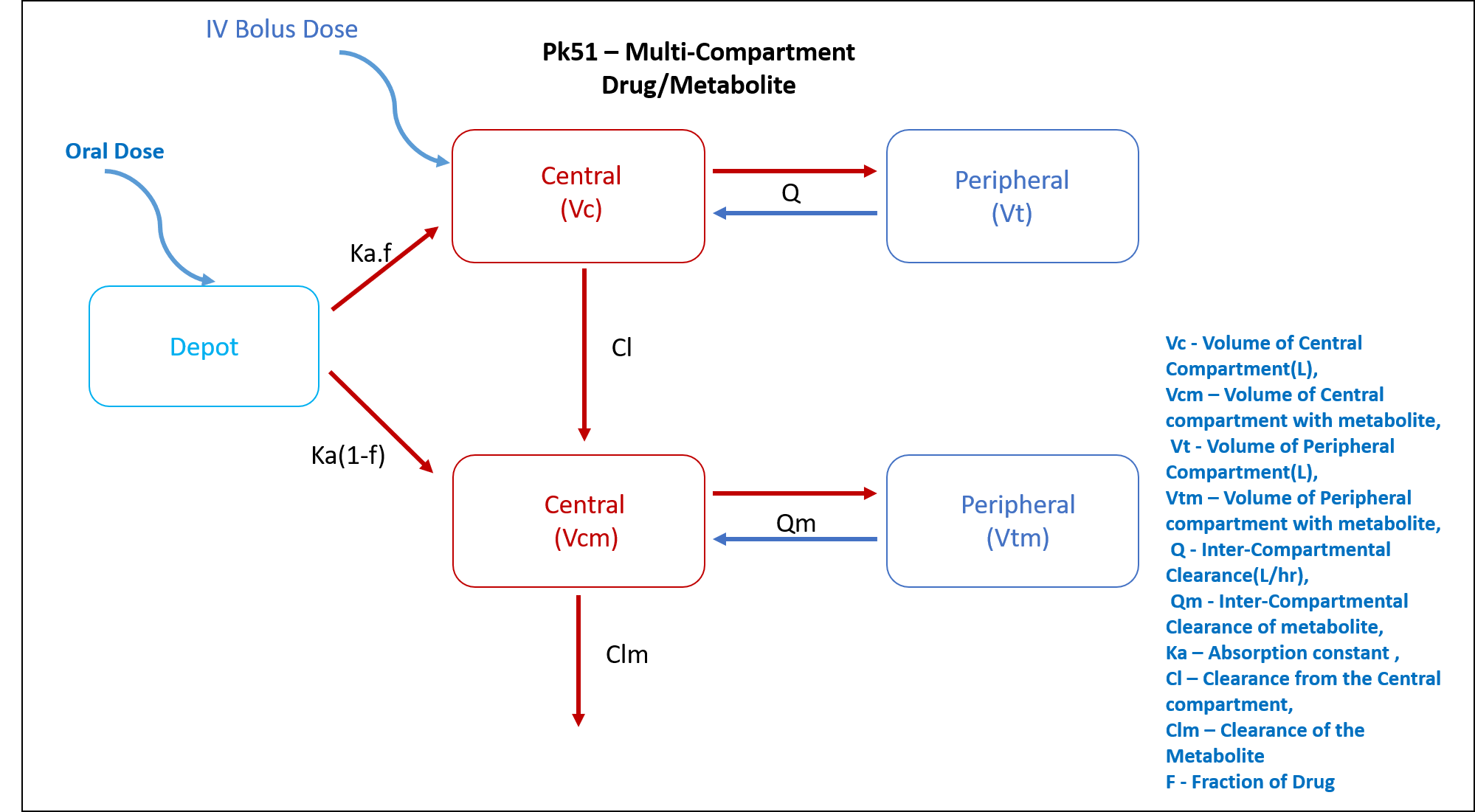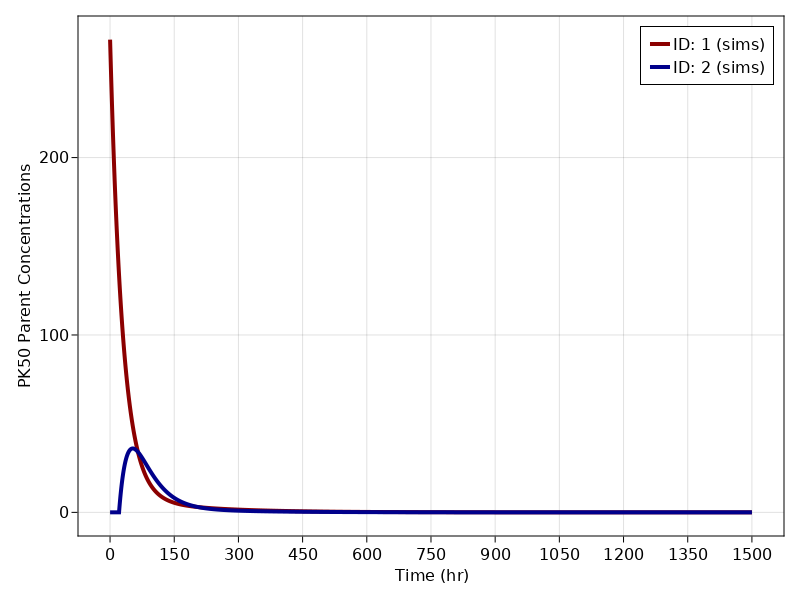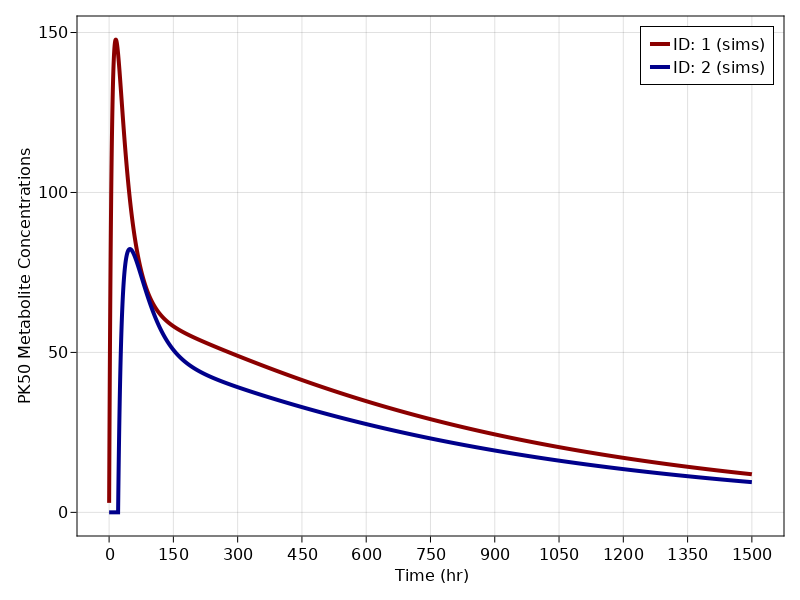# Exercise PK51 - Multi-compartment drug/metabolite

### Background

• Structural model - Multi-Compartment Drug/metabolite

• Dosage Regimen - 5000 mg IV and 8000 mg Oral

• Number of Subjects - 2### Learning Outcome

In this model, drug is administered both IV and orally at different occasions to different subjects and plasma data is collected, The concentrations are obtained for both the drugs and metabolite. This will help you learn the two compartment parent - metabolite kinetics

### Objectives

In this tutorial, you will learn how to build multi compartment drug/metabolite model and to simulate the model for different subjects and dosage regimens.

### Libraries

Call the "necessary" libraries to get start.

using Random
using Pumas
using PumasUtilities
using CairoMakie


### Model

In this one compartment model, we administer dose on oral and central compartment at two different occasions.

pk_51               = @model begin
desc            = "Two Compartment Model with Metabolite Compartment"
timeu           = u"hr"
end

@param begin
"Volume of Central Compartment (L)"
tvvc            ∈ RealDomain(lower=0)
"Clearance (L/hr)"
tvcl            ∈ RealDomain(lower=0)
"Inter Compartmental Clearance (L/hr)"
tvcld           ∈ RealDomain(lower=0)
"Volume of Peripheral Compartment (L)"
tvvt            ∈ RealDomain(lower=0)
"Volume of Central Compartment of Metabolite (L)"
tvvcm           ∈ RealDomain(lower=0)
"Clearance of metabolite (L/hr)"
tvclm           ∈ RealDomain(lower=0)
"Inter Compartmental Clearance of Metabolite (L/hr)"
tvcldm          ∈ RealDomain(lower=0)
"Volume of Peripheral Compartment of Metabolite (L)"
tvvtm           ∈ RealDomain(lower=0)
"Absorption rate constant (hr⁻¹)"
tvka            ∈ RealDomain(lower=0)
"Fraction of drug absorbed"
tvf             ∈ RealDomain(lower=0)
"Lag time (hr)"
tvlag           ∈ RealDomain(lower=0)
Ω               ∈ PDiagDomain(9)
"Proportional RUV - Plasma"
σ²_prop_cp      ∈ RealDomain(lower=0)
"Proportional RUV - Metabolite"
σ²_prop_met     ∈ RealDomain(lower=0)
end

@random begin
η               ~ MvNormal(Ω)
end

@pre begin
Vc              = tvvc * exp(η)
Cl              = tvcl * exp(η)
Cld             = tvcld * exp(η)
Vt              = tvvt * exp(η)
Vcm             = tvvcm * exp(η)
Clm             = tvclm * exp(η)
Cldm            = tvcldm * exp(η)
Vtm             = tvvtm * exp(η)
Ka              = tvka * exp(η)
end

@dosecontrol begin
bioav           = (Depot = tvf , Metabolite = (1-tvf))
lags            = (Depot = tvlag,)
end

@dynamics begin
Depot'          = -Ka*Depot
Central'        =  Ka*Depot - (Cl/Vc)*Central - (Cld/Vc)*Central + (Cld/Vt)*Peripheral
Peripheral'     = (Cld/Vc)*Central - (Cld/Vt)*Peripheral
Metabolite'     =  Ka*Depot + (Cl/Vc)*Central - (Clm/Vcm)*Metabolite - (Cldm/Vcm)*Metabolite + (Cldm/Vtm)*PeriMetabolite
PeriMetabolite' = (Cldm/Vcm)*Metabolite - (Cldm/Vtm)*PeriMetabolite
end

@derived begin
cp              = @. Central/Vc
"""
Observed Concentration (...)
"""
dv_cp           ~ @. Normal(cp, sqrt(cp^2*σ²_prop_cp))
met             = @. Metabolite/Vcm
"""
Observed Concentration (...)
"""
dv_met          ~ @. Normal(met, sqrt(met^2*σ²_prop_met))
end
end

PumasModel
Parameters: tvvc, tvcl, tvcld, tvvt, tvvcm, tvclm, tvcldm, tvvtm, tvka, t
vf, tvlag, Ω, σ²_prop_cp, σ²_prop_met
Random effects: η
Covariates:
Dynamical variables: Depot, Central, Peripheral, Metabolite, PeriMetaboli
te
Derived: cp, dv_cp, met, dv_met
Observed: cp, dv_cp, met, dv_met


### Parameters

The parameters are as given below. tv represents the typical value for parameters.

• $Cl$ - Clearance (L/hr)

• $Clm$ - Clearance of metabolite (L/hr)

• $Cld$ - Inter Compartmental Clearance (L/hr)

• $Cldm$ - Inter Compartmental Clearance of metabolite (L/hr)

• $Vc$ - Volume of Central Compartment (L)

• $Vcm$ - Volume of Central Compartment of Metabolite (L)

• $f$ - Fraction of drug absorbed

• $lags$ - Lag time (hr)

• $Ka$ - Absorption rate constant (hr⁻¹)

• $Vt$ - Volume of Peripheral Compartment (L)

• $Vtm$ - Volume of Peripheral Compartment of metabolite (L)

• $Ω$ - Between Subject Variability

• $σ$ - Residual error

param = ( tvvc        = 18.7,
tvcl        = 0.55,
tvcld       = 0.073,
tvvt        = 10,
tvvcm       = 4.9,
tvclm       = 0.08,
tvcldm      = 0.58,
tvvtm       = 55,
tvka        = 0.03,
tvf         = 0.24,
tvlag       = 21,
Ω           = Diagonal([0.0,0.0,0.0,0.0,0.0,0.0,0.0,0.0,0.0]),
σ²_prop_cp  = 0.015,
σ²_prop_met = 0.015)

(tvvc = 18.7, tvcl = 0.55, tvcld = 0.073, tvvt = 10, tvvcm = 4.9, tvclm = 0
.08, tvcldm = 0.58, tvvtm = 55, tvka = 0.03, tvf = 0.24, tvlag = 21, Ω = [0
.0 0.0 … 0.0 0.0; 0.0 0.0 … 0.0 0.0; … ; 0.0 0.0 … 0.0 0.0; 0.0 0.0 … 0.0 0
.0], σ²_prop_cp = 0.015, σ²_prop_met = 0.015)


### Dosage Regimen

##### IV

A single dose of 5000 mg given as a rapid IV injection

ev1  = DosageRegimen(5000, time = 0, cmt = 2)
sub1 = Subject(id = 1, events = ev1)

Subject
ID: 1
Events: 1

##### ORAL

A single dose of 8000 mg given orally

ev2      = DosageRegimen(8000, time=0, cmt=1)
sub2     = Subject(id = 2, events = ev2)
pop2_sub = [sub1,sub2]

Population
Subjects: 2
Observations:


### Simulation

Simulate using the simobs function.

Random.seed!(123)
sim_pop2_sub = simobs(pk_51, pop2_sub, param, obstimes = 0.1:0.1:1500)


### Visualization

f1, a1, p1 = sim_plot(pk_51, sim_pop2_sub,
observations = :cp,
color = :redsblues,
linewidth = 4,
axis = (xlabel = "Time (hr)",
ylabel = "PK50 Parent Concentrations",
xticks = 0:150:1500))
axislegend(a1)
f1f2, a2, p2 = sim_plot(pk_51, sim_pop2_sub,
observations = :met,
color = :redsblues,
linewidth = 4,
axis = (xlabel = "Time (hr)",
ylabel = "PK50 Metabolite Concentrations",
xticks = 0:150:1500))
axislegend(a2)
f2